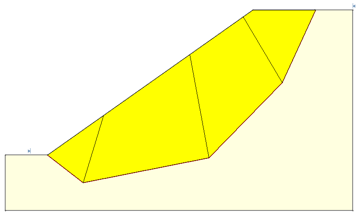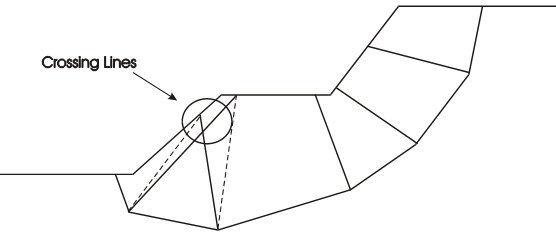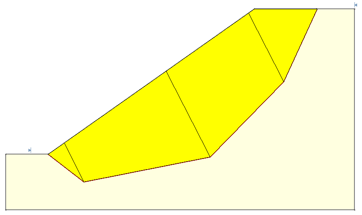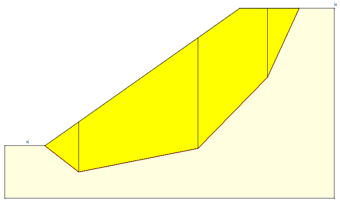Expert Modelling: Hands-on help from Rocscience Engineers. Register Here

# Sarma Slice Angles

For the Sarma Non-Vertical Slice analysis method, the factor of safety depends on the interslice angles. In the Project Settings dialog, the following options are available for defining the slice angles.

• The Optimized option will find the critical set of angles that results in the lowest factor of safety.
• Alternatively the slice angles can be determined by selecting one of the User Defined options.

NOTE: Slice Angles can also be specified for user-defined slip surfaces with the Define Slice Angles option in the Surfaces menu.

## Optimized

The interslice angles can be optimized for the Global Minimum or for All Surfaces.

Global Minimum

If this option is selected, first the factor of safety for all surfaces is calculated using bisecting angles. Then the critical set of slice angles is found only for the global minimum surface (the surface with the lowest factor of safety).Slide2 uses a multi-resolution optimization technique in order to find the set of angles that results in the lowest factor of safety. If there are weak planes in the material, slice boundaries parallel to those planes are also tested during the optimization process.

All Surfaces

If this option is selected, the slice angles for all surfaces are optimized in order to find the critical set. In most cases it is recommended to use the Global Minimum option, due to longer computational time of the All Surfaces option. However, for models with anisotropic materials (embedded weak planes) it might be necessary to optimize the slice angles of all surfaces to obtain more accurate results.

User-Defined

The interslice angles can be also determined by selecting one of the following User-Defined options.

Bisection

This option finds the bisector of the angle between the bases of two consecutive slices.

Note that if two slice boundaries cross, they will be adjusted by sorting the top locations of the slices, shown as dashed lines in the diagram below.Weighted Average Normal

If this option is selected the normal of each slice base is determined. The weighted average is calculated by scaling the angle of the normal by the length of the base of that slice. This results in a uniform interslice angle for all slices as shown below.Vertical

If the Vertical option is selected all slices will be vertical as shown in the figure below.NOTE: this option is based on the method presented by Sarma (1979) as a special case where all δ’s are set to zero. It is NOT the same as selecting Sarma under the Vertical Slices option which is based on Sarma (1973).